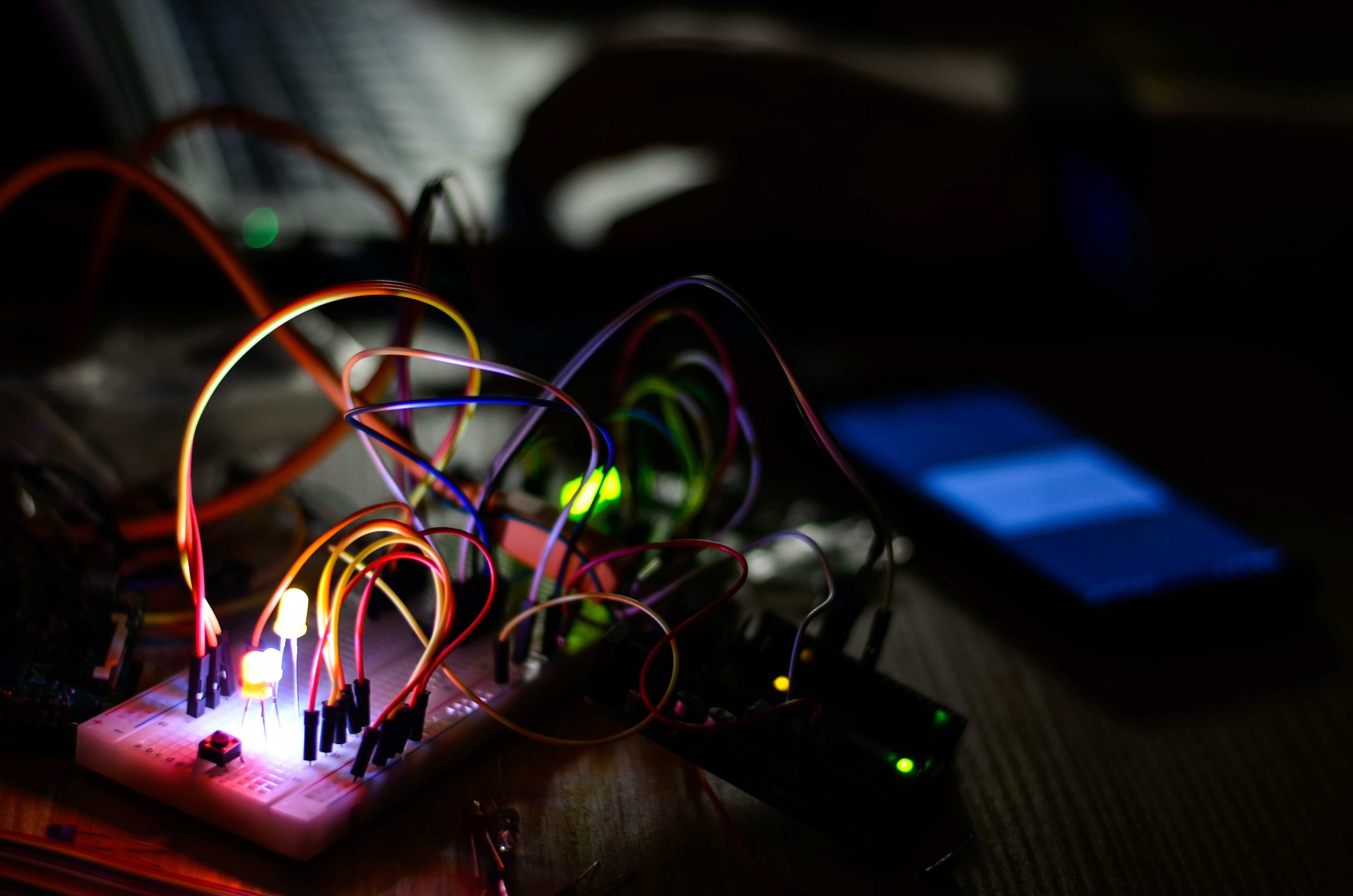Photo by Victor Aznabaev / Unsplash

# Mastering the measurement of Battery voltage with an ESP controller

IOT Nov 4, 2022

I figured out that my self-designed home devices will empty, without any sign. So I decided to create a voltage meter in my code.

# Hardware construct

For clarification, I will use an ESP device, which can only measure the largest input of 3,3V. But my Batteries have more consumption. For this, I must create first a voltage divider to get a measuring point that will supply the largest voltage.

The schematic diagram will look like this

## How do you calculate the resistor values?

First of all, here is the base Formular:

Actually, we know the Battery (lipo) input voltage is about 3,7–4 Volt (Vs), we need the largest output of 3,3 Volt (Vout).

The next step is to set the resistor (R2) this will in my case 47K Ohm. Because the higher resistor, the less current we will draw.

Now we got all values to calculate the second resistor (R2). Instead of displaying the formula, I will give you a calculator, that serves as a calculator for all your needs

In this, I put in my values, and it will calculate then the correct resistor that we need for the R1.

In my case, it calculated the value of 9,96 KOhms. I decided to use a 10KOhm resistor because this is the nearest shoppable value.

## How to measure?

After we set up the circuit, I will measure the values with an ESP Device. I will use the wemos D1 Mini for this because I got some of them on my desk for now. You can use any other device, but it must have analog input. Because we use this input for measuring the capacity of the battery.

My diagram looks like this

## The Code for getting the voltage

The code is very simple, you only must get the measurement from the Analog output sensor with the method `analogRead()` . In my example it looks like this:

``````int rawVoltage= analogRead(A0);
float fVoltage = (float)rawVoltage * 0,00381861575179
``````

I calculated the value 0,00381861575179, by dividing the voltage across the resistors with the reading from the analog input (rawVoltage).

For Example, you got in rawVoltage the value 838 and you got the measured voltage across the resistors about 3.2 Volts. Then you must calculate 3.2 /838 then you got the value 0,00381861575179, I call this the footage multiplication.

So each read from the rawVoltage from now, you must multiply it with this calculated value.

## One word on Li-ion batteries

They are very popular, but they do not discharge linearly, the discharge will look like this:

So let’s say Vfull will be 4 V and vexp will be about 3.3V, you see that this will be very rapid discharging. After this, I will hold this voltage. This will depend on your battery power. if you have more mAh then it will take longer than the batteries with lower power.

But when it finally reaches Vnom then the discharge rate picks up quickly.

## Example

If you have a 500mah battery that lasts about 4 hours in your projects and our battery reading (rawVolate) range was 3100 at full and 1700 right before it would shut down. The timeline will behave like this:

• In the first 30 minutes, you will notice the discharge vom 100% to 80%
• In the next hours, you will see a discharge from 80% to 60%
• At least you see a drop from 60% to 0% in 30 minutes.

## Code to get a percentage value from the reading

To get a better experience I created a mapper from my readings here’s my code

I use the map method, to get a value for the given analog read value. So I then tried to measure the values with and without the mapper function (with my 1200 mah lipo) and draw the values as a curve in Excel here’s my result:

The blue one is the curve without the mapping function and the orange one with the mapper. It turned out that it will look more comfortable for me to use.